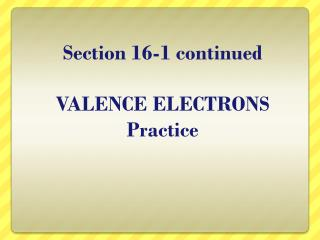# Section 16-1 continued VALENCE ELECTRONS Practice - PowerPoint PPT PresentationDownload PresentationSection 16-1 continued VALENCE ELECTRONS Practice

Section 16-1 continued VALENCE ELECTRONS PracticeDownload Presentation## Section 16-1 continued VALENCE ELECTRONS Practice

- - - - - - - - - - - - - - - - - - - - - - - - - - - E N D - - - - - - - - - - - - - - - - - - - - - - - - - - -
##### Presentation Transcript

1. Section 16-1 continuedVALENCE ELECTRONSPractice

2. Periods • Each row is called a “period” • The elements in each period have the same number of electron shells 1st Period = 1 Shell 2nd Period = 2 Shells 3rd Period = 3 Shells 4th Period = 4 Shells www.chem4kids.com

3. Groups • Each element in a group has the same number of “valence electrons” Group 8 = 8 electrons Group 1 = 1 electron Group 2 = 2 electrons 3,4,5,6,7 Except He, it has 2 electrons

4. Transition Metals • Transition Metals have slightly different rules for shells and valence electrons. • This is something you will learn about in High School Chemistry. www.chem4kids.com

5. Determine the number of shells and the number of valence electrons for: Carbon - C 2nd Period = 2 shells 4th Group = 4 valence electrons

6. Determine the number of shells and the number of valence electrons for: Sodium - Na 3rd Period = 3 shells 1st Group = 1 valence electron www.chem4kids.com

7. 10 Ne 20.18 PRACTICE? Name the element.__________ Number of protons?____ Average number of neutrons? ____ Number of shells ?____ Number of total electrons?____ Valence electrons ?_____

8. 10 Ne 20.180 Answer? Name the element. Neon Number of protons? 10 p+ Number of neutrons? 10 n Number of shells ? 2 (2nd period) Number of total electrons? 10 e- Valence electrons ? 8 ve- (8th group)

9. 4 Be 9.012 PRACTICE? Name the element.__________ Number of protons?____ Average number of neutrons? ____ Number of shells ?____ Number of total electrons?____ Valence electrons ?_____

10. 4 Be 9.012 Answer? Name the element. Beryllium Number of protons? 4 p+ Number of neutrons? 5 n Number of shells ? 2 (2nd period) Number of total electrons? 4 e- Valence electrons ? 2ve- (2nd group)

11. 16 S 32.096 PRACTICE? Name the element.__________ Number of protons?____ Average number of neutrons? ____ Number of shells ?____ Number of total electrons?____ Valence electrons ?_____

12. 16 S 32.096 Answer? Name the element. Sulfur Number of protons? 16 p+ Number of neutrons? 16 n Number of shells ? 3 (3rd period) Number of total electrons? 16 e- Valence electrons ? 6ve- (6th group)

13. 2 He 4.003 PRACTICE? Name the element.__________ Number of protons?____ Average number of neutrons? ____ Number of shells ?____ Number of total electrons?____ Valence electrons ?_____

14. 2 He 4.003 Answer? • Helium is the exception in Group 8. • Since it has just one shell, that shell can only fit 2 electrons instead of 8. • It is in this group because all the elements have a full outer shell. Name the element. Helium Number of protons? 2 p+ Number of neutrons? 2 n Number of shells ? 1 (1st period) Number of total electrons? 2 e- Valence electrons ? 2ve- (8th group - why?)